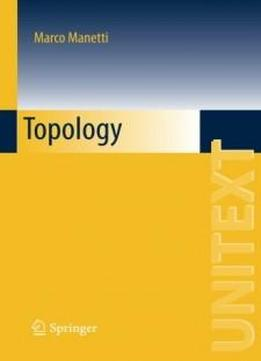# Topology (unitext) by Marco Manetti / 2015 / English / PDF

This is an introductory textbook on general and algebraic topology, aimed at anyone with a basic knowledge of calculus and linear algebra. It provides full proofs and includes many examples and exercises.

This is an introductory textbook on general and algebraic topology, aimed at anyone with a basic knowledge of calculus and linear algebra. It provides full proofs and includes many examples and exercises. The covered topics include: set theory and cardinal arithmetic; axiom of choice and Zorn's lemma; topological spaces and continuous functions; connectedness and compactness; Alexandrov compactification; quotient topologies; countability and separation axioms; prebasis and Alexander's theorem; the Tychonoff theorem and paracompactness; complete metric spaces and function spaces; Baire spaces; homotopy of maps; the fundamental group; the van Kampen theorem; covering spaces; Brouwer and Borsuk's theorems; free groups and free product of groups; and basic category theory. While it is very concrete at the beginning, abstract concepts are gradually introduced. It is suitable for anyone needing a basic, comprehensive introduction to general and algebraic topology and its applications.

The covered topics include: set theory and cardinal arithmetic; axiom of choice and Zorn's lemma; topological spaces and continuous functions; connectedness and compactness; Alexandrov compactification; quotient topologies; countability and separation axioms; prebasis and Alexander's theorem; the Tychonoff theorem and paracompactness; complete metric spaces and function spaces; Baire spaces; homotopy of maps; the fundamental group; the van Kampen theorem; covering spaces; Brouwer and Borsuk's theorems; free groups and free product of groups; and basic category theory. While it is very concrete at the beginning, abstract concepts are gradually introduced. It is suitable for anyone needing a basic, comprehensive introduction to general and algebraic topology and its applications.

views: 525